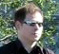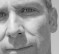# Least-squares magic bullets? The Moore-Penrose Pseudoinverse

Hello,

the topic of this brief article is a tool that can be applied to a variety of problems: The Moore-Penrose Pseudoinverse.
While maybe not exactly a magic bullet, it gives us least-squares optimal solutions, and that is under many circumstances the best we can reasonably expect.

I'll demonstrate its use on a short example. More details can be found for example on Wikipedia, or the Matlab documentation for pinv.

The complete Matlab example is here (the link opens a new window).
The purpose of this blog entry is to provide additional information to the example program.

### Problem

This picture may look more familar to electronics engineers than we'd like: The blue trace could be a measured signal that has picked up power line hum.The corresponding variable in the Matlab example is measuredSignal, a column vector with one entry per measured sample.

We'd like to reconstruct the hum signal from the measured signal (red trace), based on the knowledge of possible hum waveforms.

### Setting up the equation system

do know the exact frequency of the power line hum and its harmonics, but I do not know amplitude and phase of each term.
First, I set up a matrix with my "basis" waveforms: index=[1:m]' is a column vector with the sample numbers. The resulting basis matrix has one row per sample, and one column for each of the six candidate waveforms.### Moore-Penrose Pseudoinverse

Next, I calculate the Moore-Penrose Pseudoinverse using pinv(basis), and take a matrix-vector product with the measured signal.
As a result I get a column vector with one coefficient per basis waveform. These coefficients are my least-squares solution, fitting the known waveforms to the original signal:Next, I reconstruct the hum signal by weighting each basis waveform with its least-squares coefficient and adding the weighted waveforms:What remains is to subtract the reconstructed hum signal from the measured data.
Voilà, here is the corrected signal:The picture shows:

• The ideal, undisturbed signal
• The remainder, after subtracting reconstructedHum from measuredSignal
• The difference with regard to the ideal signal (error)

Even though the signal was completely overwhelmed by power line hum in the first picture, we were able to remove the hum almost completely. Not too bad...

For interpretation: The processing has removed as much as possible of the signal energy (sum of squares) by scaling the known waveforms. Or in other words: The remaining signal is the component of the input signal that cannot be "explained" by the basis waveforms.

Of course, if the ideal signal has components that look like power line hum ("can be explained by the basis waveforms"), those will also be removed.

The accuracy increases with the square root of the number of independent samples m. For example, to reduce the error by 3 (10) dB, it takes 4 (100) times more samples.

On a modern PC, surprisingly large problems can be handled.
A Moore-Penrose Pseudoinverse is a computationally expensive operation. But note that it is applied to the basis waveforms, not the signal. In order to process many signals of the same (or shorter) length, it needs to be computed only once.
Applying it to the measured signal involves only one "dot" (scalar) product between two vectors per coefficient.

Thanks for reading! I hope the example will be useful.

Complete Matlab example, ready to run: here

[ - ]
Comment by October 1, 2014Markus, in your example we do not need to use pseudoinverse, because rank(basis) = #columns. So, left inverse of such matrix always exists. The usual way: inv(basis' * basis) * basis' * measuredData is enough.

But we have to use pseudoinverse, if we add columns into matrix, which are linear combinations of original columns. In this case, matrix rank will stay 6, while number of columns will be bigger. For such matrix there is no way to use typical way and pseudoinverse is required.
[ - ]
Comment by October 1, 2014Hi Denis,

thank you for the comment.

When you write
>> "The usual way: inv(basis' * basis) * basis' * measuredData "
this already puts it out of reach of most "practical" engineers, who aren't DSP-specialized, and/or have left their "math IV" exam behind a long time ago.

No, my intention with this article - which isn't paying any attention at all to linear algebra - is to advertise the method as a general problem solving tool. It is particularly attractive because a large class of problems can be solved with a common pattern. It may be inefficient, but I wouldn't see this as a problem in offline calculations. Instead, "one size fits all" is the main selling point. Also, the pinv-equation or the similar "magic backslash operator" in Matlab are easy to remember.

Someone implementing the same on limited resources would need to spend more time with the calculation, decide what is really necessary and how can it be done efficiently. But that is clearly not the target audience here.
[ - ]
Comment by October 2, 2014Markus, well in general case, I agree, backslash is enough and we don't even need to mention pseudoinverse.
Practically, I can't remember any DSP-model, where columns might be linearly dependent and pseudoinverse is needed. Do you know any examples?
[ - ]
Comment by October 23, 2010Nice Markus! By the way, even thought the code section is not officially live yet, you can go ahead and link towards your code snippet: http://www.dsprelated.com/showcode/4.php
[ - ]
Comment by October 24, 2010Thanks, done!
[ - ]
Comment by November 2, 2010Thanks for your kind introduction. I think that '3' have to be changed to '5' in the equation of 'hum5th' in matlab example code. If so, the pictures will be changed.
[ - ]
Comment by November 2, 2010Hello, It -is- a mistake, thank you very much. I'll fix it later today and take new screenshots. In the example as it is right now, the artificially generated noise term contains only a 3rd harmonic, but the 5th is missing.
[ - ]
Comment by January 18, 2012please let me know the how design the desired sgnal [d(n)] in LMS algorithm
[ - ]
Comment by October 3, 2014Actually, Matlab's "backslash" and MP-Pseudoinverse differ for redundant basis vectors. One minimizes the norm of the result, the other uses the smallest number of components (details: http://www.mathworks.se/help/matlab/ref/pinv.html)

>> I agree, backslash is enough and we don't even need to mention pseudoinverse.
Coming soon: The sequel, titled "LS Episode II: The Backslash".
I bet it will attract readers out of sheer curiosity :)

To post reply to a comment, click on the 'reply' button attached to each comment. To post a new comment (not a reply to a comment) check out the 'Write a Comment' tab at the top of the comments.